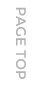## News

Home / News / How to calculate the thickness of notebook custom back

### How to calculate the thickness of notebook custom back

Whether the theoretical data is consistent with the actual value depends on the error between the manufacturing thickness value of each sheet of paper and the theoretical value. This issue should be understood as follows:
The more pages a notebook has, the greater the error accumulated throughout the notebook. Therefore, we can't take the theoretical thickness of the paper provided by the paper factory as the criterion, and we must measure the product. It is to actually measure the thickness of the white paper as the basis for the thickness of a single sheet of paper.
When actually measuring the white paper, it is necessary to use the large to reduce the error. For example, it is best to measure 2 reams of paper and reduce the measured value by 1000 times. In order to prevent the error between batches of products, it is necessary to measure several more pieces of paper and take the average value. Every time the paper of the same gram weight is fed, it is necessary to measure, accumulate data, and correct the original value to make it infinitely close to the actual value.
In order to reduce the measurement and batch product error of a single sheet of paper, the most accurate method is to bind out a sample of white paper. Of course, it is best to draw paper from several pieces of white paper.
Since the printed notebook page is thicker than the white paper, use a printed notebook to bind out the sample. In this case, the difference between the thickness of the notebook and the thickness of the actual batch notebook will be less.
More accurate notebook back thickness, only the actual sample notebook can be bound with each printed notebook page. But even the samples made in this way are unlikely to be exactly the same as the batch notebooks. This requires designers to understand and think of ways.
Theoretical Calculation Method of Laptop Back Thickness
1. Calculation of the thickness of the back of a general paperback notebook
In theory, the thickness of the notebook back of a notebook is generally equal to the thickness of the notebook. The thickness of the notebook should be equal to the sum of the thickness of all notebook pages of the notebook. If the notebook is only printed on the same paper, then,
Notebook thickness = thickness value of a piece of paper × number of sheets (or a number of pages/2).
If more than two kinds of paper are used for printing, the thickness of each paper can be calculated by the above formula, and then the thickness of each paper can be added up.
2. Calculation of back thickness of the hardcover notebook
1) Square (flat) back hardcover notebook back thickness = the paperback (notebook core) thickness of this notebook + the thickness of the front and rear ring linings (the thickness of each ring lining paper × 4) + the thickness of the cardboard (the thickness of each cardboard × 4) 2) + The thickness of the notebook shell fabric (the thickness of each fabric × 2).
2) The thickness of the round-back hardcover notebook actually refers to the length of the round-back arc of the hardcover notebook. Generally, the circular potential of this arc is 120 degrees, and the chord corresponding to this arc is the straight line thickness (square back thickness) of this hardcover notebook. The ratio of chord and arc at this time is 1:1.15.